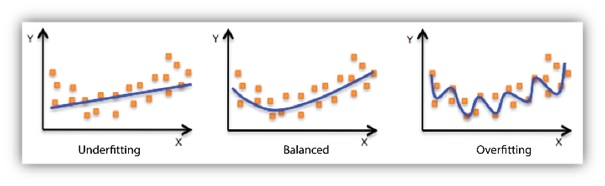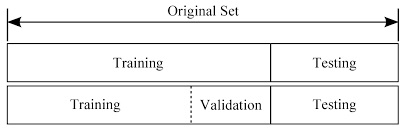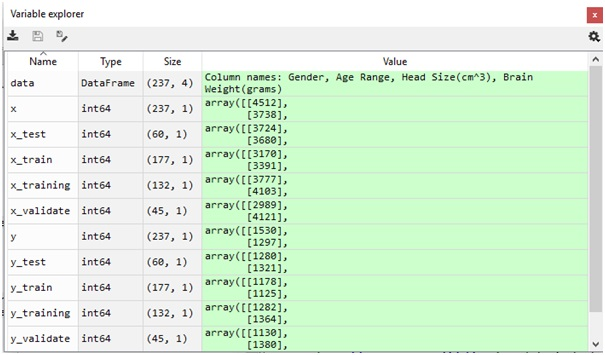# Validation Before Testing in Machine Learning

In this tutorial, we will learn about the validation before resting in machine learning, why it is required, and how to create a validation data set using Python? By Raunak Goswami Last updated : April 16, 2023

In my previous article, we have discussed about the need to train and test our model and we wrote a code to split the given data into training and test sets.

## What is the need of validation before testing?

Before moving to the validation portion, we need to see what is the need to use validation procedure before performing the testing procedure in the given data set. At times when we are dealing with a huge amount of data there is a certain chance that maybe the data used by our model during learning produced a biased result and in this case as we use the test set to check the accuracy of our model the following 2 cases can arise:

1. Under fitting of the test data
2. Over fitting of the test dataImage source: https://docs.aws.amazon.com/machine-learning/latest/dg/images/mlconcepts_image5.png

So then how do we deal with such a problem? Well, the answer is pretty simple if we can somehow use a 3rd data set to validate the results obtained from the training set so that we can adjust the various hyperparameters like learning rate and batch values to get a balanced result on the validation set which will, in turn, increase the accuracy of our model in estimating the target values from the test set.Image source: https://rpubs.com/charlydethibault/348566

Here, you can see that the validation set is nothing but a subset of the training data set that we create. Here do remember that when we create a partition from a dataset. The data present in the datasets are shuffled randomly to remove biased results.

So, let us write a simple code to create a validation data set in python:

## Python code to create a validation data set

```# -*- coding: utf-8 -*-
"""
Created on Wed Aug  1 22:18:11 2018

@author: Raunak Goswami
"""

import numpy as np
import pandas as pd
import matplotlib.pyplot as plt

"""here the directory of my code and the headbrain.csv
file is same make sure both the files are stored in the same folder
or directory"""

#this will show the first five records of the whole data

#this will create a variable x which has the feature values i.e brain weight
x=data.iloc[:,2:3].values
#this will create a variable y which has the target value i.e brain weight
y=data.iloc[:,3:4].values

#splitting the data into training and test
"""
the following statement written below will split x and y into 2 parts:
1.training variables named x_train and y_train
2.test variables named x_test and y_test
The splitting will be done in the ratio of 1:4 as we have mentioned
the test_size as 1/4 of the total size
"""
from sklearn.cross_validation import train_test_split
x_train,x_test,y_train,y_test=train_test_split(x,y,test_size=1/4,random_state=0)

#Here we again split the training data further
##into training and validating sets.
#observe that the size of the validating set is
#1/4 of the training set and not of the whole dataset
from sklearn.cross_validation import train_test_split
x_training,x_validate,y_training,y_validate=train_test_split(x_train,y_train,test_size=1/4,random_state=0)
```

After running this python code on your Spyder tool provided by the Anaconda distribution just cross check your variable explorer:On the image above you can see that we have split the train variables into training variables and validate variables.